# 一、什么是编译（理解）

## 1.1 计算机程序设计语言及编译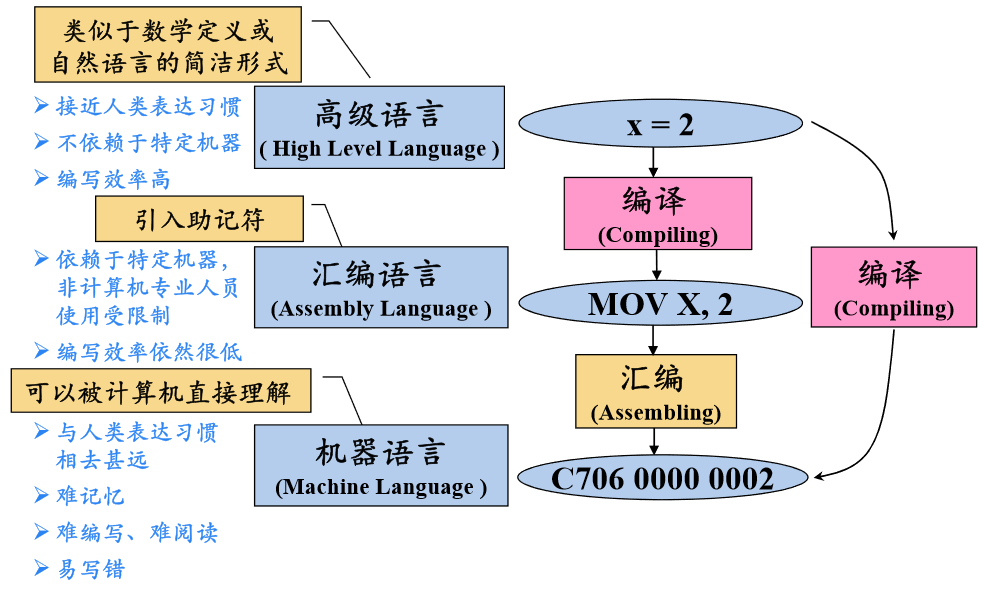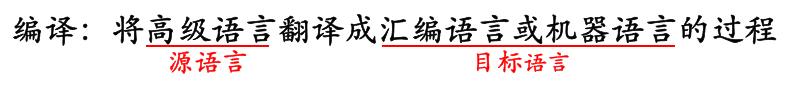## 1.2 编译器在语言处理系统中的位置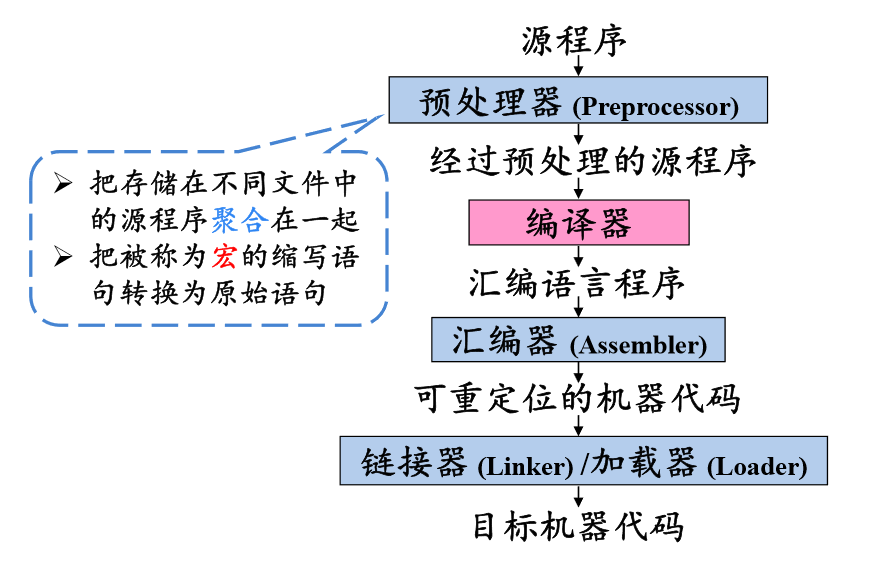## 1.3 编译系统的结构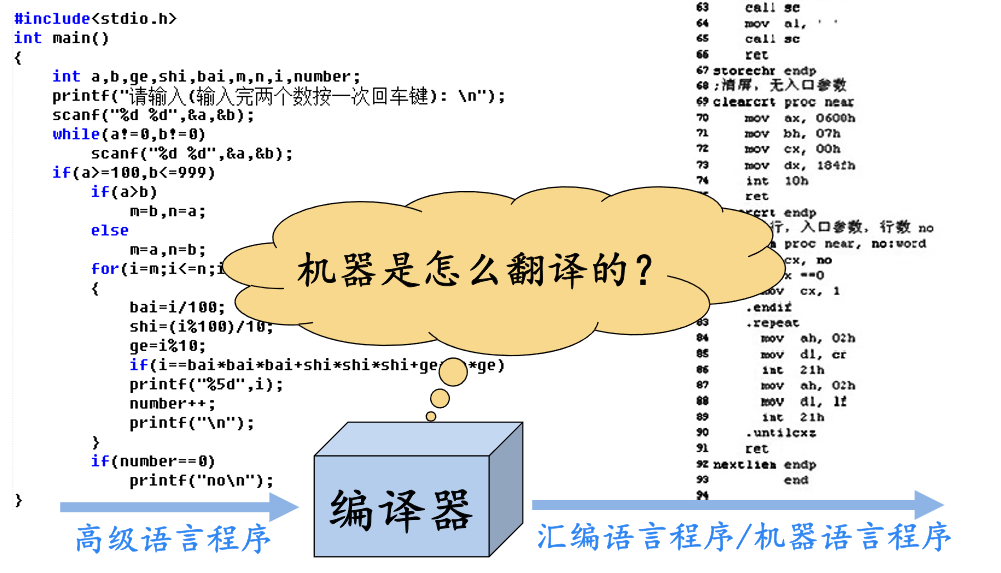## 1.4 人工英汉翻译的例子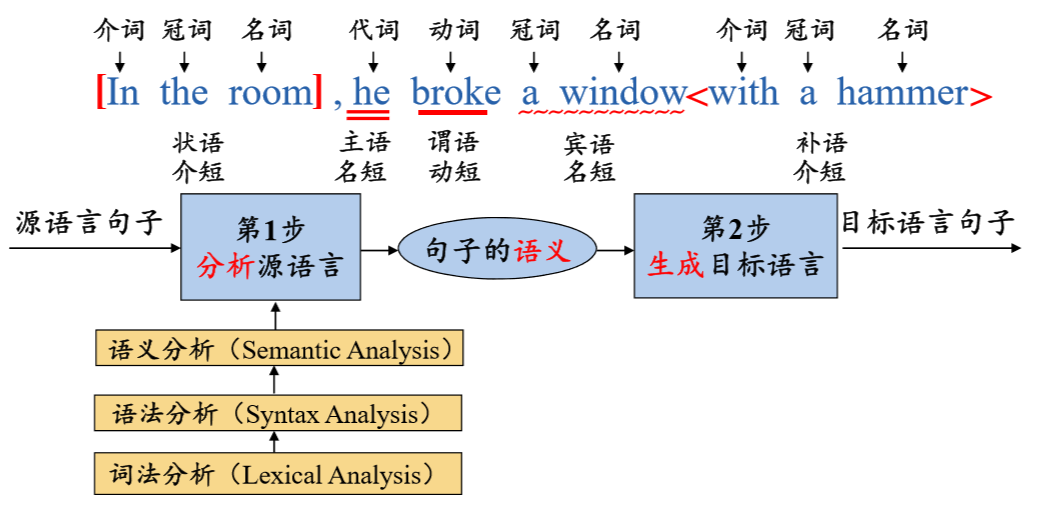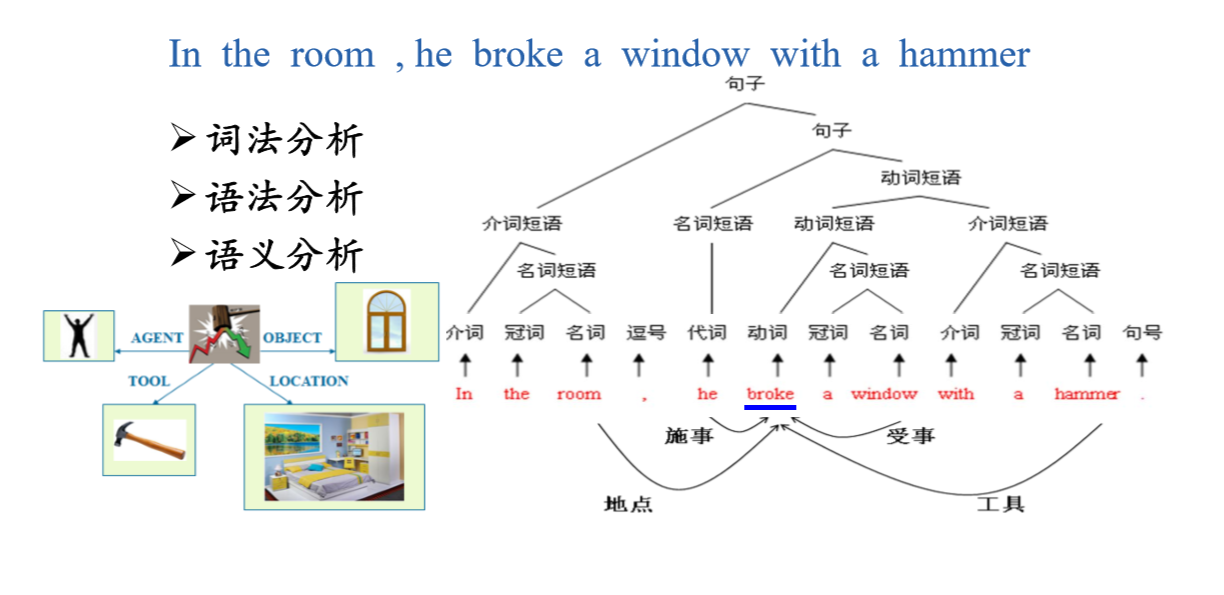## 1.5 编译器的结构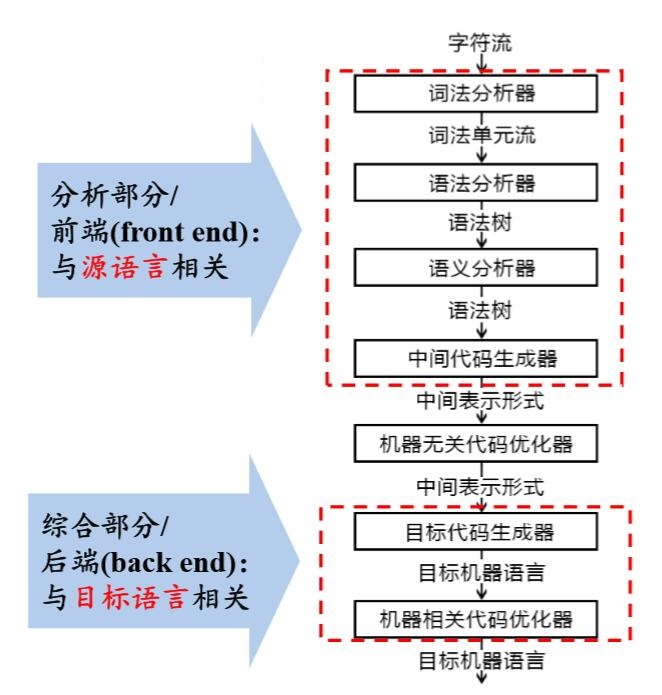# 二、文法（掌握）

• 认识终结符和非终结符
• 文法的类型
• 判断一个串是否为某个文法的句型

## 2.1 认识终结符和非终结符

``````有文法G2[S]为：
S->Ap
S->Bq
A->a
A->cA
B->b
B->dB``````

## 2.2 文法的类型

### 2.2.1 几种文法的理解

• 0型文法

• 1型文法

1型文法又称上下有关文法，在0型文法的基础上每一个α->β，都有|β|>=|α|，这里的|β|表示 β的长度。

• 2型文法

2型文法又称上下文无关文法，，它对应下推自动机，2型文法是在1型文法的基础上再满足，每一个α->β都有α是非终结符。

• 3型文法

3型文法又称正规文法，它对应有限状态自动机，它是在2型文法的基础上再满足，A->a|aB（右线性）或者

A->a|Ba（左线性）。

``````A->ε|aB
B->Ab|a``````

1、我们分开来写，应该是：A->ε A->aB B->Ab B->a
2、我们先来判断是否符合0型文法：0型文法规定左边必须有非终结符，那么这些都是符合的。
3、我们再来看是否符合1型文法：1型文法规定从小推到大，也符合。
4、我们再来看是否符合2型文法：2型文法规定左边必须是非终结符，也满足。
5、我们继续看是否符合3型文法：规定只能符合右线性或者左线性，那么前面一个应该是符合右线性的，后面一个是符合左线性的。所以综合起来就不符合3型文法了。

### 2.2.2 几种文法的关系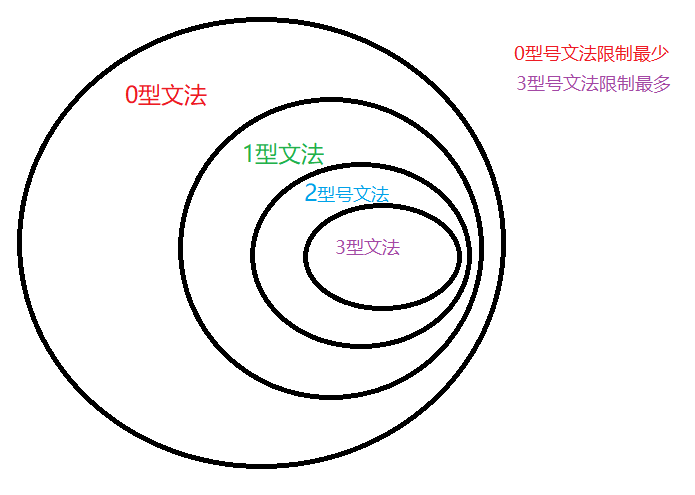# 三、正规式（掌握）

## 3.1 正规式与正规文法之间的转换

``````|       | 文法产生式 | 正规式 |
| ----- | ---------- | ------ |
| 规则1 | A->xB,B->y | A=xy   |
| 规则2 | A->xA|y   | A=x^*y |
| 规则3 | A->x,A->y  | A=x|y |``````

``````文法G[S]:S->xSx|y所描述的语言是_____（n>=0）
A.(xyx)^*
B.xyx^*
C.xy^*x
D.x^*yx^*``````

``````语言L=｛a^mb^n|m>=0,n>=1｝的正则表达式是_____。
A.a^*bb^*
B.aa^*bb^*
C.aa^*b^*
D.a^*b^*``````

# 四、有限自动机（掌握）

• NFA与DFA的定义
• NFA转化为DFA
• 正规表达式与有限自动机之间的转化

## 4.1 NFA与DFA的定义

### 4.1.1确定的有限自动机（DFA）的定义

M=（S，∑，f，S0，Z）

S是一个有限状态集合

∑是一个字母表，它的每个元素称为一个输入字符

f是一个从S✖∑至S的单值部分映射，f（S，a）=s‘ 意味着：当现行状态为s，输入字符为a时，将状态到下一状态s‘。我们称为s‘为s的一个后继状态。

S0∈S，是唯一的初态。

Z⊆S，是一个终态集。

``````DFA=({S,A,B,C,f},{1,0},F,S,{f}),为了不混淆，F在下方用K表示。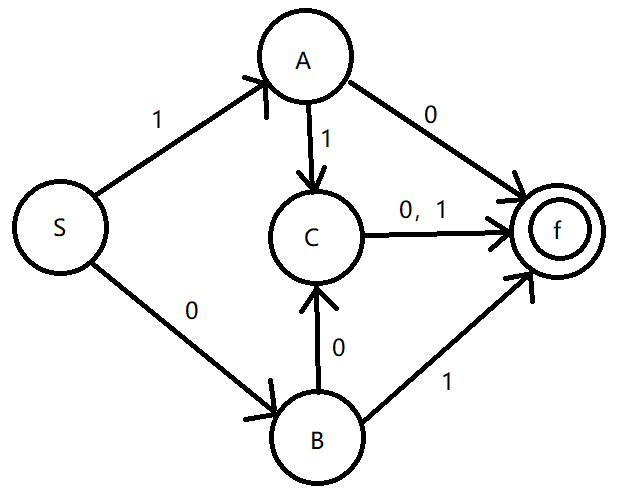### 4.1.2 不确定的有限自动机（NFA）的定义

M=（S，∑，f，S0，Z）

S是一个有限状态集合

∑是一个字母表，它的每个元素称为一个输入字符

f是一个从S✖∑至S的单值部分映射，f（S，a）=s‘ 意味着：当现行状态为s，输入字符为a时，将状态到下一状态s‘。我们称为s‘为s的一个后继状态。

S0⊆S，是一个非空初态集。

Z⊆S，是一个终态集。

## 4.2 NFA转化为DFA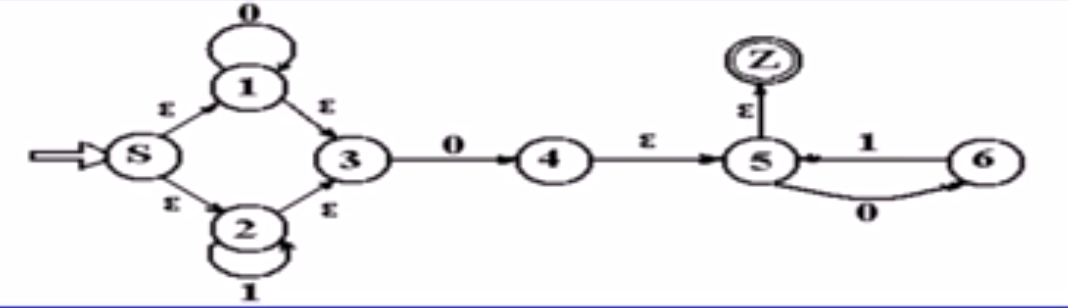II0I1
{S,1,2,3}{1,3,4,5,Z}{2,3}
{1,3,4,5,Z}T1T3
{2,3}{4,5,Z}{2,3}
T2{6}T3
T1{1,3,4,5,6,Z}{5,Z}
{6}T3{5,Z}
{5,Z}{6}T3

``````（1）A.2   B.4   C.3   D.5
（2）A.1,3,4,5,Z   B.2,3   C.6   D.4,5,Z
（3）A.{Z}   B.{6}   C.{4,5,Z}   D.{}``````

A；D；D

## 4.3 正规式与有限自动机之间的转换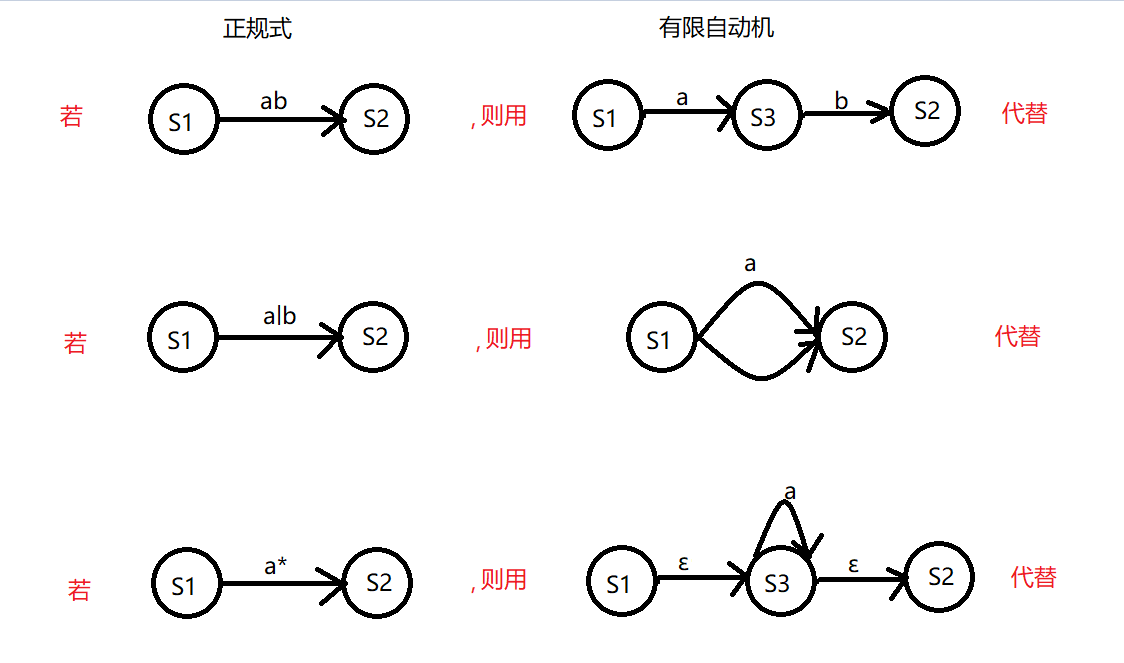``(_|a)(_|a|d)^*``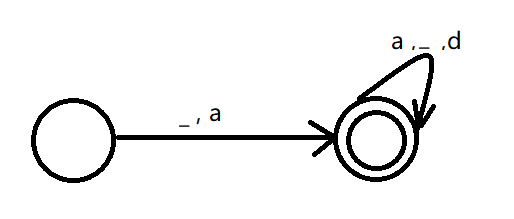``````A．0*|(0|1)0
B．(0|10)*
C．0*((0|1)0)*
D．0* (10)*``````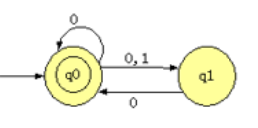# 五、语法推导树（掌握）

## 5.1 语法树

1. 每个结点都有一个标记，此标记是V的一个符号；
2. 根的标记是S；
3. 若一个结点n至少有一个它自己除外的子孙，并且有标记A，则A肯定在Vn中；
4. 如果结点n的直接子孙，从左到右的次序是结点n1,n2...nk,其标记分别是：A1,A2...Ak，那么A->A1,A2...Ak，一定是P中的一个产生式。

``````若文法G={{a,b},{S,A},S,P},其中：S->aAS|a; A->SbA|SS|ba;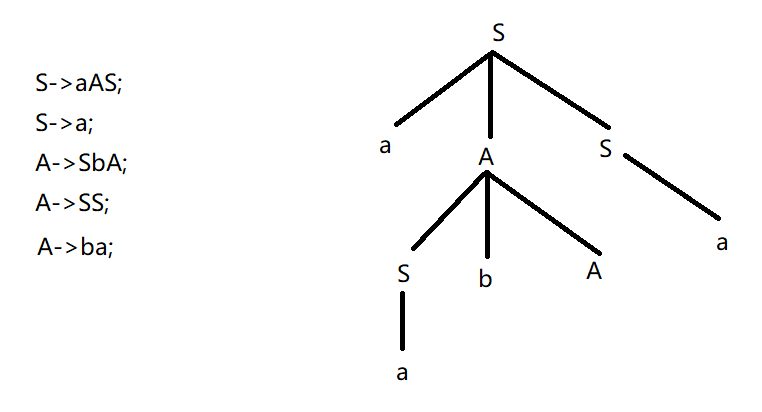## 5.2 短语、简单短语、句柄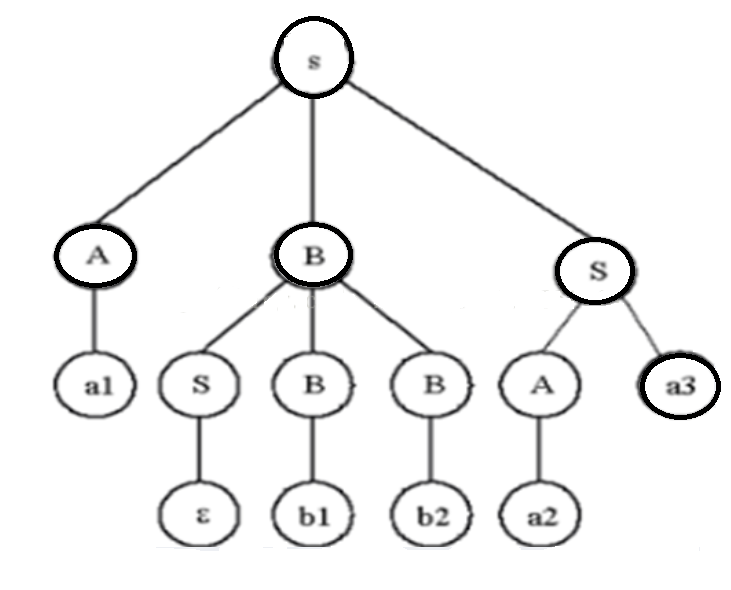# 六、LL(1)文法（掌握）

• 判断是否是LL(1)文法
• 求生成式的FIRST、FOLLOW、SELECT集合

## 6.1 什么是LL(1)文法

（1）如果α、β均不能推导出ε，则 FIRST(α) ∩ FIRST(β) = ∅。

（2）α 和 β 至多有一个能推导出 ε。

（3）如果 β *═> ε，则 FIRST(α) ∩ FOLLOW(A) = ∅。

## 6.2 求FIRST集合

``````1.如果X是一个终结符号，那么FIRST(X) = X。

2.如果X是一个非终结符号，且X -> Y1Y2 …Yk是一个产生式，其中k ≥ 1，那么如果对于某个i , a 在FIRST(Yi)中且ε在所有的FIRST(Y1)、FIRST(Y2)、….、FIRST(Yi-1)中，就把a加入到FIRST(X)中。也就是说，Y1…Yi-1 =>* ε。如果多于所有的j = 1,2,3,..,k , ε在FIRST(Yj)中，那么将 ε 加入到FIRST(X)中。比如，FIRST(Y1)中的所有符号一定在FIRST(X)中。如果Y1 不能推导出 ε ，那么，我们就不会再向FIRST(X)中加入任何符号，但是如果Y1 =>* ε ，那么我们就加上FIRST(Y2)，以此类推。

3.如果X -> ε 是一个产生式，那么将ε 加入到FIRST(X)中。``````

``````E → TE'
E' → +TE' |ε
T → FT '
T' → *FT ' |ε
F → (E)|id``````

FIRST(E): 由E -> TE’可得FIRST(E) = FIRST(T)
FIRST(T): 由T -> FT’ 可得FIRST(T) = FIRST(F)
FIRST(F): 由F -> (E)和F -> id 可得FIRST(F) = { ( , id };
FIRST(T’): 由T’-> FT’ 和 T’-> ε 可得FIRST(T’) = { , ε };
FIRST(E’): 由E’-> +TE’ 和 E’-> ε可得FIRST(E’) = { + , ε };

FIRST ( E ) = { ( id }
FIRST ( E' ) = { + ε }
FIRST ( T ) = { ( id }
FIRST ( T' ) = { * ε }
FIRST ( F ) = { ( id }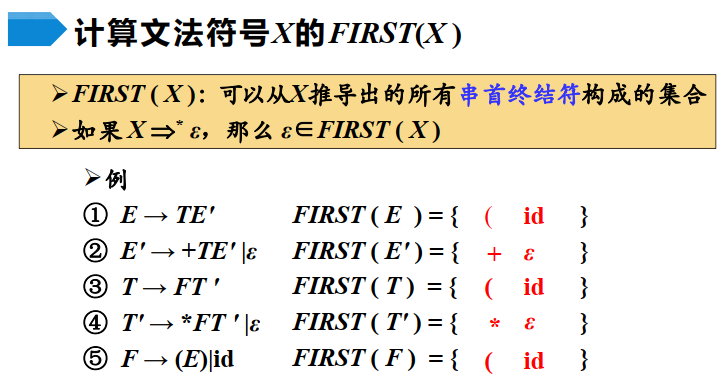## 6.3 求FOLLOW集合

``````1.将 \$ 放到FOLLOW(S)中，其中S是开始符号，而 \$ 是输入右端的结束标记。

2.如果存在一个产生式 A -> αBβ ， 那么FIRST(β)中除 ε之外的所有符号都在FOLLOW(B)中。

3.如果存在一个产生式A -> αB，或存在产生式 A -> αBβ 且 FIRST(β)包含 ε ，那么FOLLOW(A)中的所有符号都在FOLLOW(B)中。``````

``````E → TE'
E' → +TE' |ε
T → FT '
T' → *FT ' |ε
F → (E)|id``````

FOLLOW(E): 由F -> (E)可得，FOLLOW(E) = { ) ，\$}
FOLLOW(E’): 由E -> TE’ 和 E’-> +TE’ 可得，FOLLOW(E’) = FOLLOW(E)
FOLLOW(T): 由E -> TE’、E’-> +TE’ 和 E’-> ε 可得，FOLLOW(T) = FIRST(E’) / ε +FOLLOW(E) + FOLLOE(E’ )
FOLLOW(T’): 由T -> FT’ 和 T’-> *FT’ 可得，FOLLOW(T’) = FOLLOW(T)
FOLLOW(F): 由T -> FT’、T’-> *FT’ 和 T’-> ε 可得，FOLLOW(F) = FIRST(T’) / ε +FOLLOW(T) + FOLLOW(T’)

FOLLOW(E) = { ) ，\$}
FOLLOW(E’) = FOLLOW(E) = { ) ，\$}
FOLLOW(T) = FIRST(E’) / ε +FOLLOW(E) + FOLLOE(E’) = {+ , ) , \$}
FOLLOW(T’) = FOLLOW(T) = {+ , ) , \$}
FOLLOW(F) = FIRST(T’) / ε +FOLLOW(T) + FOLLOW(T’) = {* , + , ) , \$}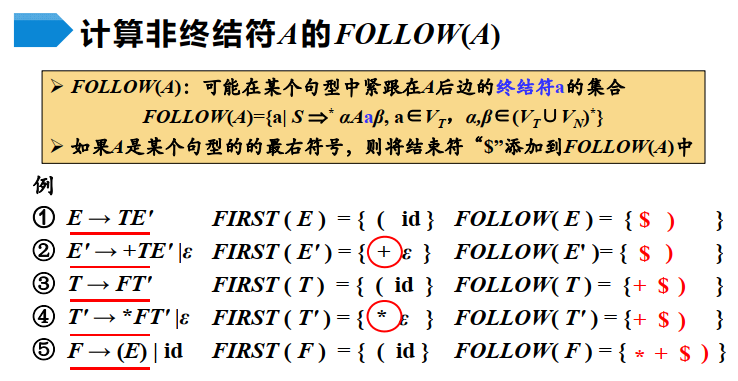## 6.4 求SELECT集合

``````1.如果ε∉FIRST（a），那么SELECT（A→a）=FIRST（a）

2.如果ε∈EFIRST（a），那么SELECT（A→a）=（FIRST（a）-{}）U FOLLOW（A）``````

``````(1) E → T E '
(2) E '→ + T E '
(3) E '→ ε
(4) T → F T '
(5) T ' → * F T '
(6) T ' → ε
(7) F → ( E )
(8) F → id``````

XFIRST( X )FOLLOW( X )
E( id\$ )
E '+ ε\$ )
T( id+ ) \$
T '* ε+ ) \$
F( id* + ) \$

SELECT (1)= { ( id }
SELECT (2)= { + }
SELECT (3)= { \$ ) }
SELECT (4)= { ( id }
SELECT (5)= { * }
SELECT (6)= { + ) \$ }
SELECT (7)= { ( }
SELECT (8)= { id }

<待更新...>

CSDN博文《原来编译原理可以这么学》